# STEP BY STEP HOW TO RUN DRILLING SURGING / SWABBING PRESSURE CALCULATION

## What Is Definition Of The Surge / Swab Pressure In Drilling & Who To CalculateDifference Between Surging And Swabbing In Drilling

### Drilling Surge /Swab Pressure Definition

Surge pressures describe pressure changes in the annulus resulting from pipe movement. As the drill pipe is pulled from the well, mud flows down the annulus to fill the void left by the pipe. As the drill pipe is lowered into the well, mud is forced out of the flow line. Pressure changes caused by lowering the pipe into the well are called surge pressures and are generally considered to be added to the hydrostatic pressure.

### What Is The Risks / Effects For Increasing Surge / Swab Pressures While drilling?

Removing drill pipe from the well creates swab pressures, which are negative, resulting in a net lowering of pressure in the well. Many problems are caused by swab and surge pressures. Removing the drill pipe at rates that create large swab pressures can induce a kick by lowering the wellbore pressure below formation pressure which will lead to a well control issue. Surge pressures increase the total wellbore pressure and can cause formation fracturing and lost circulation.

### Is It Difficult To Calculate Swab / Surge Pressure ?Fig.1 Annular fluid flow resulting from pipe movement

Computing swab and surge pressures is difficult because of the manner in which the fluid flows as pipe is moved in the well. As shown in Fig. 1, drill pipe moving down the annulus causes the mud adjacent to the pipe to be dragged downward. Since the annulus is a fixed volume, however, and the mud is considered incompressible, some drilling fluids must flow out of the annulus. The mechanics are different than pumping since the fluid flow is considered to be only one direction.

### How Could Burkhardt build his model to calculate Swab / Surge Pressure while drilling?

Burkhardt conducted field studies to evaluate surge and swab pressures. His work involved running casing into a drilled well that was equipped with pressure sensors. A typical pressure response to the pipe movement is shown on Fig. 2. Positive and negative pressures are shown for the cases of pipe being lifted off the slips and later being decelerated.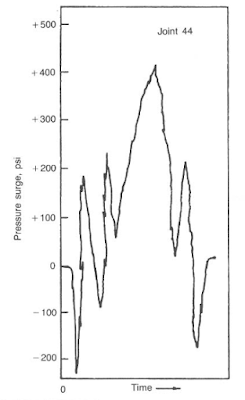Fig.2 Typical pressure-surge pattern measured as a joint of casing was lowered into the wellbore

The complex fluid flow model shown in Fig.3 was evaluated by Burkhardt. Using involved mathematics, Burkhardt developed a relationship between pipe and hole geometries and the effect of the dragging mud adjacent to the pipe. The term clinging constant represents this relationship (Fig.4)Fig.3Fig.4 Mud clinging constant, K, relative to annulus geometries

The mud velocity in the annulus must be computed before the clinging constant can be applied. The flow rate of steel for a closed drill string into the well is given by Eq.1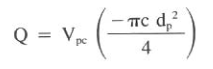Eq.1
Where:
Q = flow rate, gal/min
Vp = pipe velocity,ft/sec

The velocity in the annulus is the quotient of flow rate and area:Eq.2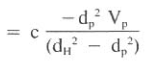Eq.3
If the pipe is open-ended, the flow velocity is solved in a similar manner:Eq.4

Applying the clinging constant, k, the effective annular velocity (Vc) is as follows: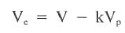Eq.5
Burkhardt noted that clinging constant (k) = 0.45 was a good assumption for most typical geometries.

In operation, a pipe velocity is used to compute the flow of pipe into the well, which is assumed to be equal to flow out of the well. The clinging constant is applied to yield an effective velocity based on the complex flow patterns in the annulus. The surge or swab pressures are computed by substituting the effective velocity into any of the previously defined friction pressure equations. It is reasonable to use laminar flow equations since normal pipe velocities seldom cause greater than critical velocities.

### Example on how to run Surge / Swab Pressure Calculations

A well is experiencing lost circulation problems at the bottom of the casing string. A liner will be run into the well. If the liner is lowered at a maximum rate of 93 ft/min, will the surge pressures exceed the fracture gradient? Use the Bingham model and assume laminar annular flow. In addition, assume
the peak pipe velocity is the same as the average value.

casing depth = 10,000 ft, casing ID = 8.5 in.
open hole depth = 13,000 ft, open hole OD = 8.5 in.
liner size = 7 in. (flush joint), liner length = 3,600 ft, drillpipe = 4.5 in.
mud = 16.6 Ib/gal
= 38 cp (PV)
= 15 Ib/lOOfe (YP)
pipe velocity = 93 ft/min
= 1.55 ft/sec

(Assume the liner has a closed end from a float shoe.)

Solution:

1. The maximum surge pressures occur when the bottom of the liner reaches the casing seat (see Fig. 5).

2. The mud flow rate leaving the well when the liner shoe reaches the casing seat is:
V = Q / 2.448 (d^2)
Q =  2.448 V (d^2) = 2.448 (1.55 ft/sec) (4.5 in.)^2
Q = 76.8 gal/min

3. Compute the annular velocities around the drillpipe (Vap)and the liner (Val)

Vap = Q / 2.448 (dH^2- dP^2) = 76.8/ 2.448 (8.5^2- 4.5^2)
= 0.603 ft/sec = 36 ft/min

Val= Q / 2.448 (dH^2- dP^2) = 76.8/ 2.448 (8.5^2- 7^2)= 1.349 ft/sec = 80.9 ft/min

4. Refer to Fig. 4 and determine the clinging constant, k, for the pipe and liner:

pipe - ratio = 4.5/8.5 = 0.529
k 0.38
liner - ratio = 7/8.5 = 0.823
k 0.45Fig.4 Mud clinging constant, K, relative to annulus geometries

5. The effective annular velocities around the pipe (Vpe)and the liner (Vle) are:
Vpe = V - 0.38 Vp = (0.603) - (0.38) x ( - 1.55) = 1.192 ft/sec
Vle = V - 0.45 Vp = (1.349 ft/sec) - (0.45) x( - 1.55) = 2.046 ft/sec

6. The pressure surge caused by the drill pipe using Bingham model for Laminar flow is as follows (use Eq.6):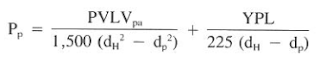Eq.6
Pp = {(38)(6,400)(1.192) / 1,500 (8.5^2 - 4.5^2 )} + {(15)(6,400) / 225 (8.5-4.5)}
Pp = 3.716 psi + 106 psi = 110 psi

7. The pressure surge caused by the liner is:
Pl = {(38)(3,600)(2.046) / 1,500 (8.5^2 - 7^2 )} + {(15)(3,600) / 225 (8.5-7)}
Pl =8.0 psi + 160 psi = 168 psi

8. The total pressure surge and equivalent mud weight is:

110 psi + 168 psi = 278 psi
EMW = pressure x 19.323 / depth + mud weight
EMW = 278 psi x 19.23 / 10000 ft + 16.6 lb/gal = 17.13 lb/gal

9. Therefore, the fracture gradient of 17.0 Ib/gal would be exceeded (17.1 Ib/gal) at a pipe velocity of 93 ft/min.

10. In reality, the flow regime in the annulus was turbulent opposite the liner. The assumption of laminar flow in this example was done for simplicity and illustration

### Notes About Surging & Swabbing Pressures Calculations

Open-ended pipe increases the complexity of the calculations considerably. Pressure drops in the annulus and drillstring must be equal. Since different geometries exist, however, flow rates in the annulus and pipe will not be the same. In addition, it is possible that the annulus will be laminar, while fluid flow in the pipe will be turbulent. A trial-and-error solution is used by assuming a major percentage of the flow will be up the annulus with the remainder in the pipe. From these assumed volumes, pressure losses are computed and compared and the volumes are adjusted, depending on the computed pressure relationships. If the first trial was a 75:25 annulus to pipe ratio and a pressure distribution of 200:300 psi was observed, a second trial might be at 80:20. The procedure is iterated until a ratio is obtained that yields equal pressure drops in both the pipe and annulus.

In a practical sense, surge and swab pressures are composed of the pressures required to break the gels in the mud and the pressures caused by actual fluid movement. Thick muds can often produce such high gels that surge pressure resulting from this component are significant. The yield point and gel strength of the mud affect this type of pressure.

### What Is The Maximum Allowable surging Pressure & Maximum Allowable Swabbing Pressure

If a maximum allowable swab or surge pressure is known, it is possible to determine a maximum pulling or running speed for the pipe. The maximum surge pressure is usually the difference between the lowest exposed fracture gradient and the mud weight. The maximum swab pressure is the difference between the greatest formation pressure from an exposed permeable zone and the mud weight. The swab pressure equations are solved in reverse order to arrive at a pipe velocity.

### Example On How To Calculate Maximum running or pulling velocity in drilling oilfield

Refer to the data in above Example. If the drilling engineer at the well site does not wish to exceed a 16.9-1b/gal equivalent surge pressure, what is the maximum velocity that the pipe can be run into the well?

Solution:
1. Determine the maximum allowable surge pressure so the 16.6-1b/gal mud does not exceed the 16.9-1b/gal equivalent value:

maximum allowable surge pressure = 0.052 x (16.9 - 16.6) x(10,000ft) = 156 psi

2. The 156-psi surge pressure is the maximum amount that can be generated by the friction pressure opposite the pipe and liner, or:
156 shall be lower than or equal Pp+ Pl

3. Substituting the pressure equations for Pp & Pl:
{ (38) (6,400) (Vpe) / 1.500 (8.5^2 - 4.5^2 )  + (15) (6,400) / 225 (8.5^2- 4.5^2) } +  { (38) (3,600) (Vle) / 1.500(8.5^2 - 7^2 )  + (15)(3,600) / 225 (8.5- 7) }

=  3.118 Vpe + 3.922 Vle+ 266 psi

156 psi shall be lower than or equal { 3.118 Vpe + 3.922 Vle+ 266 psi } which can be rewrite as following

-110 psi shall be lower than or equal { 3.118 Vpe + 3.922 Vle }

4. Since the sum of the velocity components is a negative number, the pipe cannot be run into the well at any velocity without exceeding the 16.9Ib/gal limitation. The yield point component of the pressure surge will cause the pressures to exceed the 156- psi value.